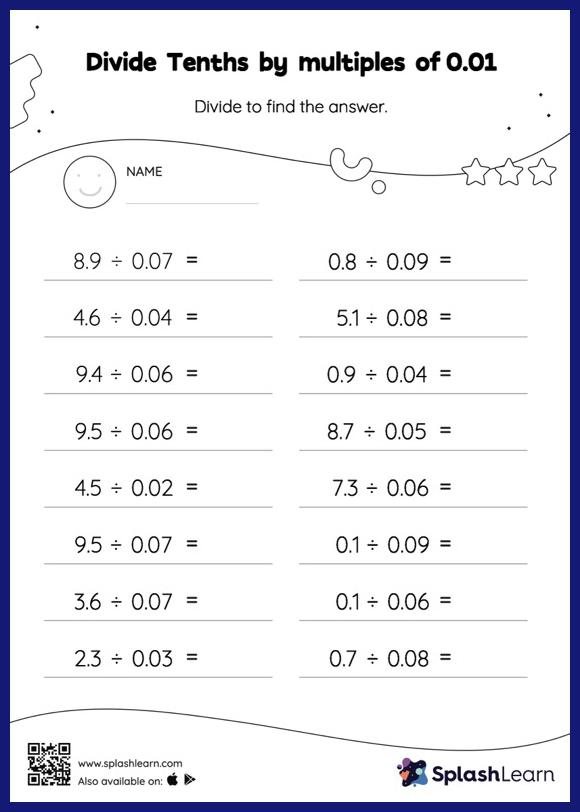# Divide Tenths by multiples of 0.01 with Remainder: Horizontal Division Worksheet

Home > Divide Tenths by multiples of 0.01 with Remainder: Horizontal DivisionGive your little one the opportunity to dive deeper into division with this divide tenths by multiples of 0.01 With remainder worksheet. When students divide two decimals, they first divide as if there is no decimal. Depending on the number of decimal digits in each number, they add a decimal point at the appropriate location in the quotient. Students practice this concept in great detail with this divide tenths by multiples of 0.01 With remainder worksheet. In this worksheet, students practice solving problems written in the horizontal format. How numbers are laid out in a problem affects the method a student employs to solve it. Therefore to develop actual fluency and mastery of multiple strategies, students must practice different formats.